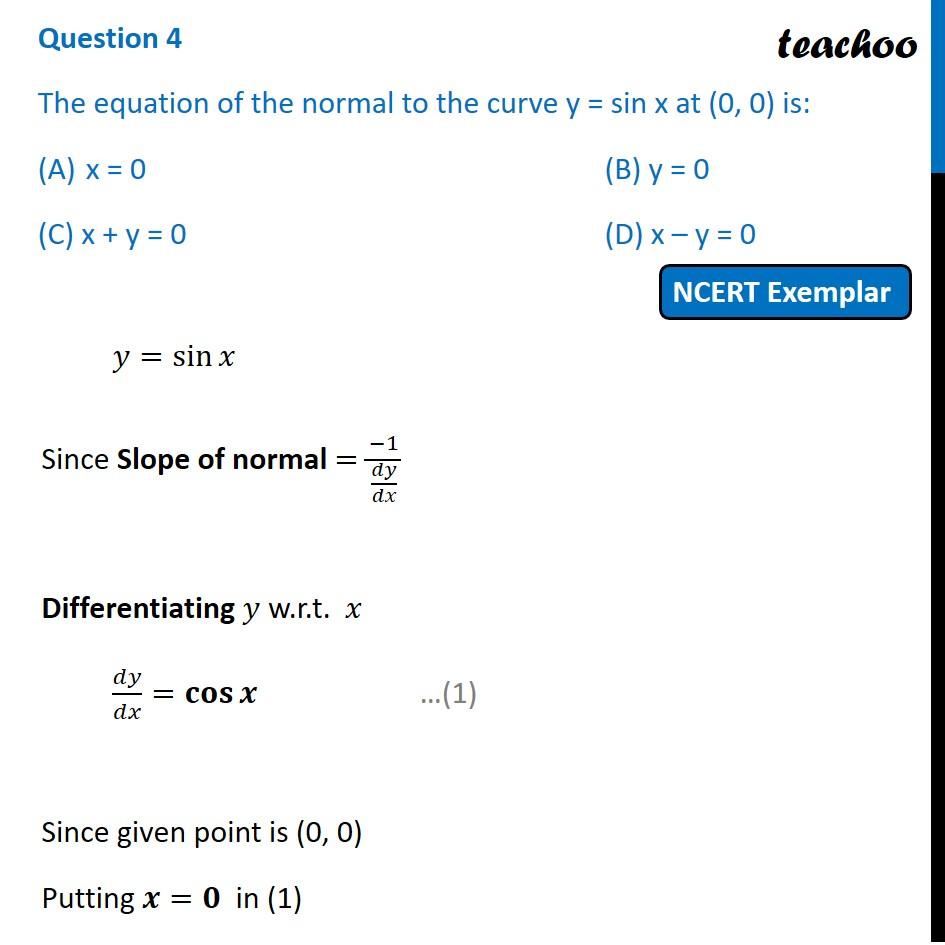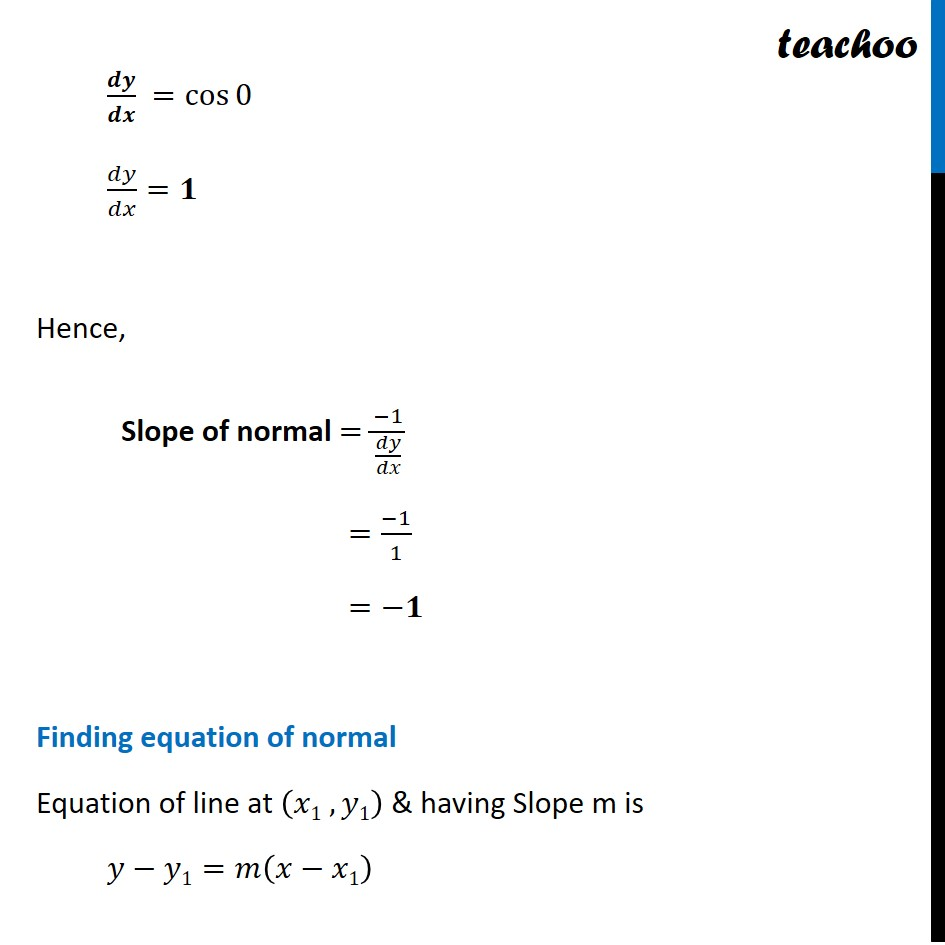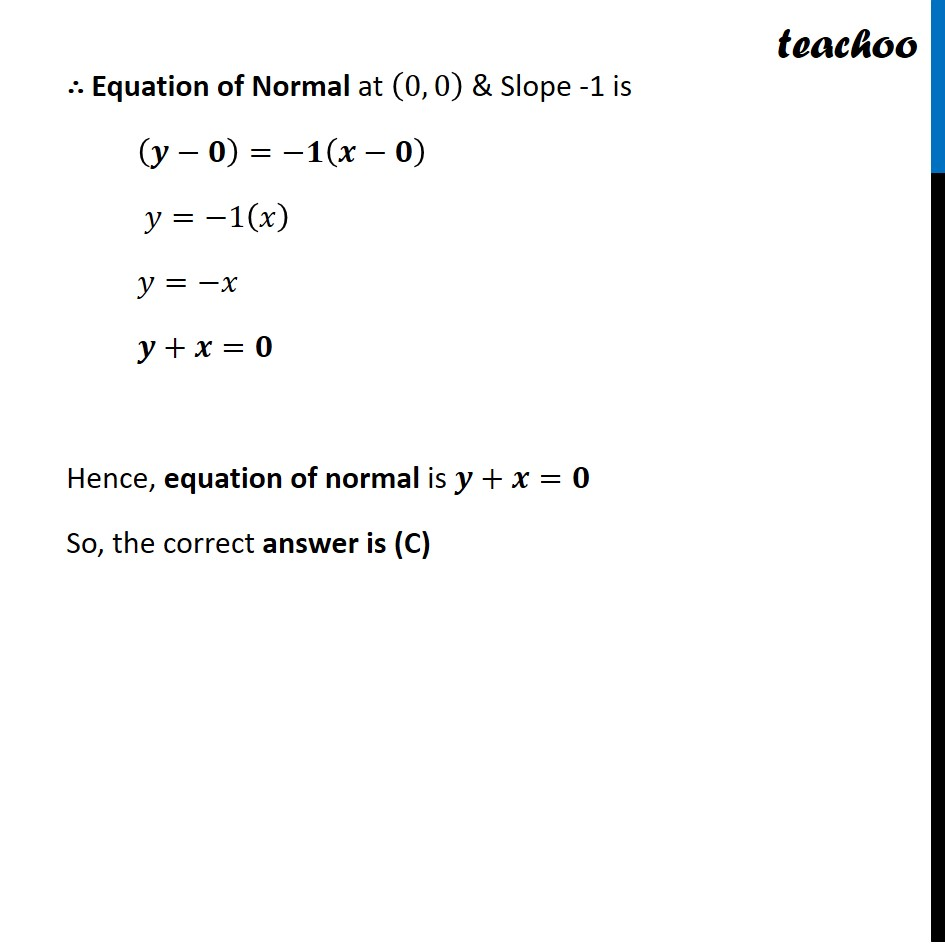NCERT Exemplar - MCQs

Chapter 6 Class 12 Application of Derivatives
Serial order wise

## (C) x + y = 0           (D) x – y = 0

This question is similar to Ex 6.3, 14 (i) - Chapter 6 Class 12 - Application of DerivativesLearn in your speed, with individual attention - Teachoo Maths 1-on-1 Class

### Transcript

Question 4 The equation of the normal to the curve y = sin x at (0, 0) is: x = 0 (B) y = 0 (C) x + y = 0 (D) x – y = 0 𝑦=sin⁡𝑥 Since Slope of normal =(−1)/(𝑑𝑦/𝑑𝑥) Differentiating 𝑦 w.r.t. 𝑥 𝑑𝑦/𝑑𝑥=𝐜𝐨𝐬⁡𝒙 Since given point is (0, 0) Putting 𝒙=𝟎 in (1) 𝒅𝒚/𝒅𝒙 =cos⁡0 𝑑𝑦/𝑑𝑥=𝟏 Hence, Slope of normal =(−1)/(𝑑𝑦/𝑑𝑥) =(−1)/1 =−𝟏 Finding equation of normal Equation of line at (𝑥1 , 𝑦1) & having Slope m is 𝑦−𝑦1=𝑚(𝑥−𝑥1) ∴ Equation of Normal at (0, 0) & Slope -1 is (𝒚−𝟎)=−𝟏(𝒙−𝟎) 𝑦=−1(𝑥) 𝑦=−𝑥 𝒚+𝒙=𝟎 Hence, equation of normal is 𝒚+𝒙=𝟎 So, the correct answer is (C)